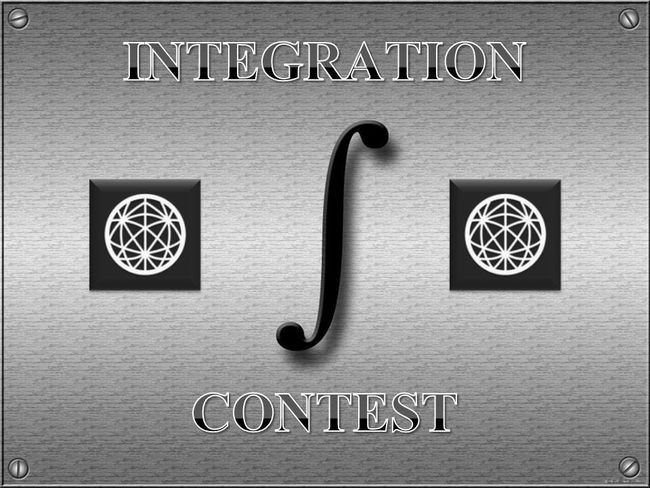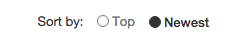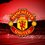# Brilliant Integration Contest - Season 3 (Part 2)Hi Brilliant! Due to many problems in part 1 it had become slow to load. So this is a sequel of Brilliant Integration - Season 3(Part 1)

The aims of the Integration contest are to improve skills in the computation of integrals, to learn from each other as much as possible, and of course to have fun. Anyone here may participate in this contest.

The rules are as follows:

• I will start by posting the first problem. If there is a user solves it, then they must post a new one.

• You may only post a solution of the problem below the thread of problem and post your proposed problem in a new thread. Put them separately.

• Only make substantial comment that will contribute to the discussion.

• Make sure you know how to solve your own problem before posting it in case there is no one can answer it within 48 hours, then you must post the solution and you have a right to post another problem.

• If the one who solves the last problem does not post his/her own problem after solving it within a day, then the one who has a right to post a problem is the last solver before him/her.

• The scope of questions is only computation of integrals either definite or indefinite integrals.

• You are NOT allowed to post a multiple integrals problem.

• It is NOT compulsory to post original problems. But make sure it has not been posted on brilliant.

• Do not copy questions from last year's contest. If anyone found to do so he/she will be banned from taking further part in this contest

Format your post is as follows:

 1 2 3 4 5 6 7 **SOLUTION OF PROBLEM xxx (number of problem) :** **[Post your solution here]** **PROBLEM xxx (number of problem) :** **[Post your problem here]** 

The comments will be easiest to follow if you sort by "Newest":4 years, 7 months ago

This discussion board is a place to discuss our Daily Challenges and the math and science related to those challenges. Explanations are more than just a solution — they should explain the steps and thinking strategies that you used to obtain the solution. Comments should further the discussion of math and science.

When posting on Brilliant:

• Use the emojis to react to an explanation, whether you're congratulating a job well done , or just really confused .
• Ask specific questions about the challenge or the steps in somebody's explanation. Well-posed questions can add a lot to the discussion, but posting "I don't understand!" doesn't help anyone.
• Try to contribute something new to the discussion, whether it is an extension, generalization or other idea related to the challenge.

MarkdownAppears as
*italics* or _italics_ italics
**bold** or __bold__ bold
- bulleted- list
• bulleted
• list
1. numbered2. list
1. numbered
2. list
Note: you must add a full line of space before and after lists for them to show up correctly
paragraph 1paragraph 2

paragraph 1

paragraph 2

[example link](https://brilliant.org)example link
> This is a quote
This is a quote
    # I indented these lines
# 4 spaces, and now they show
# up as a code block.

print "hello world"
# I indented these lines
# 4 spaces, and now they show
# up as a code block.

print "hello world"
MathAppears as
Remember to wrap math in $$ ... $$ or $ ... $ to ensure proper formatting.
2 \times 3 $2 \times 3$
2^{34} $2^{34}$
a_{i-1} $a_{i-1}$
\frac{2}{3} $\frac{2}{3}$
\sqrt{2} $\sqrt{2}$
\sum_{i=1}^3 $\sum_{i=1}^3$
\sin \theta $\sin \theta$
\boxed{123} $\boxed{123}$

Sort by:

Problem 26 :

Show that $\int_0^\infty \ln\left(\frac{a^s + x^s}{b^s + x^s}\right)\,dx \; =\; \pi(a-b)\mathrm{cosec}\tfrac{\pi}{s} \hspace{1cm} a,b > 0\,,\,s > 1 \;.$

This problem has been solved by Fdp Dpf.

- 4 years, 7 months ago

Solution problem 26:

Let , $\displaystyle F(a)=\int_0^{+\infty} \ln\left(\dfrac{a^s+x^s}{b^s+x^s}\right)dx$

Observe that, $F(b)=0$

$\displaystyle F^\prime(a)=sa^{s-1}\int_0^{+\infty} \dfrac{1}{a^s+x^s}dx$

Perform the change of variable,

$y=\dfrac{x}{a}$,

$\displaystyle F^\prime(a)=s\int_0^{+\infty} \dfrac{1}{1+x^s}dx$

It's well known that,

$\displaystyle \int_0^{+\infty} \dfrac{1}{1+x^s}dx=\dfrac{\pi}{s}\mathrm{cosec}\left(\dfrac{\pi}{s}\right)$

Therefore,

$\displaystyle F^\prime(a)=\pi\mathrm{cosec}\left(\dfrac{\pi}{s}\right)$

Therefore, $F(a)=\pi\mathrm{cosec}\left(\dfrac{\pi}{s}\right)a+k$ ,

k a real constant.

Since $F(b)=0$ then $k=-\pi\mathrm{cosec}\left(\dfrac{\pi}{s}\right)b$

Therefore,

$\boxedF(a)=\pi (a-b)\mathrm{cosec}\left(\dfrac{\pi}{s}\right)$

Proof of:

$\displaystyle \int_0^{+\infty} \dfrac{1}{1+x^s}dx=\dfrac{\pi}{s}\mathrm{cosec}\left(\dfrac{\pi}{s}\right)$

Perform the change of variable

$y=x^s$

$\displaystyle \int_0^{+\infty} \dfrac{1}{1+x^s}dx=\dfrac{1}{s} \int_0^{+\infty} \dfrac{x^{\tfrac{1}{s}-1}}{1+x}dx$

\displaystyle \begin{aligned}\int_0^{+\infty} \dfrac{x^{\tfrac{1}{s}-1}}{1+x}dx&=\beta\left(\dfrac{1}{s},1-\dfrac{1}{s}\right)\\ &=\dfrac{\Gamma\left(\dfrac{1}{s}\right)\Gamma\left(1-\dfrac{1}{s}\right)}{\Gamma(1)}\\ &= \dfrac{\pi}{\sin\left(\dfrac{\pi}{s}\right)}\\ &=\pi\mathrm{cosec}\left(\dfrac{\pi}{s}\right) \end{aligned}

$\beta$ being the Beta Euler function. Third line is the use of Euler's reflection formula.

- 4 years, 7 months ago

Problem 25 :

Prove That

$\dfrac{1}{\pi} \int_{0}^{\pi} x \arctan\left( \dfrac{p \sin x}{1+p \cos x} \right) \ \mathrm{d}x = \operatorname{Li}_{2}(p) \quad \forall \ |p| <1$

Notation : $\operatorname{Li}_{2}(z)$ denotes the Dilogarithm Function.

This problem has been solved by Mark Hennings.

- 4 years, 7 months ago

If we define $g(p) \; = \; \int_0^\pi \ln(1 + 2p \cos x + p^2)\,dx \hspace{1cm} |p| < 1$ then $g(0) = 0$ and, using the complex subsitution $z = e^{ix}$, \begin{aligned} g'(p) & = \int_0^\pi \frac{2\cos x + 2p}{1 + 2p\cos x + p^2}\,dx \; = \; \frac12\int_{-\pi}^\pi \frac{2\cos x + 2p}{1 + 2p\cos x + p^2}\,dx \\ & = \frac{1}{2}\int_{|z|=1} \frac{z + z^{-1} + 2p}{1 + p(z + z^{-1}) + p^2} \frac{dz}{iz} \; = \; \frac{1}{2i}\int_{|z|=1} \frac{z^2 + 2pz + 1}{z(pz+1)(z+p)}\,dz \\ & = \pi\left(\mathrm{Res}_{z=0} + \mathrm{Res}_{z=-p}\right)\frac{z^2 + 2pz + 1}{z(pz+1)(z+p)} \\ & = \pi\left(\frac{1}{p} - \frac{1-p^2}{p(1-p^2)}\right) \; = \; 0 \end{aligned} so that $g(p) = 0$ for all $|p| < 1$. If we now define $f(p) \; = \; \frac{1}{\pi}\int_0^\pi x \tan^{-1}\left(\frac{p \sin x}{1 + p \cos x}\right)\,dx \hspace{1cm} |p| < 1$ then $f(0) = 0$ and \begin{aligned} f'(p) & = \frac{1}{\pi}\int_0^\pi \frac{x}{1 + \left(\frac{p \sin x}{1 + p\cos x}\right)^2} \times \frac{\sin x}{(1 + p\cos x)^2}\,dx \\ & = \frac{1}{\pi}\int_0^\pi \frac{x \sin x}{1 + 2p\cos x + p^2}\,dx \\ & = \frac{1}{\pi}\Big[-\frac{x}{2p}\ln(1 + 2p\cos x + p^2)\Big]_0^\pi + \frac{1}{2\pi p}\int_0^\pi \ln(1 + 2p\cos x + p^2)\,dx \\ & = -\frac{\ln(1-p)}{p} + g(p) \; = \; -\frac{\ln(1-p)}{p} \end{aligned} and hence it follows that $f(p) \,=\, \mathrm{Li}_2(p)$.

- 4 years, 7 months ago

Aliter,

Let $f(p) = \dfrac{1}{\pi}\int_{0}^{\pi} x \arctan\left( \dfrac{p\sin x}{1+ p\cos x} \right) \ \mathrm{d}x$

Firstly, we have $f(0) = 0$

Note that,

$\displaystyle \sum_{k=1}^{\infty} (-p)^{k-1} \sin (kx) = \dfrac{1}{p}\Im \left( \sum_{k=0}^{\infty} (-p e^{ix})^{k} \right)$

$\displaystyle = \dfrac{1}{p} \Im \left( \dfrac{1}{1+p e^{ix}} \right)$

$\displaystyle = \dfrac{\sin x}{p^2 +2p \cos x +1}$

where $\Im(z)$ denotes the imaginary part of $z$.

So we have,

$\displaystyle f'(p) = \dfrac{1}{\pi} \int_{0}^{\pi} \dfrac{x \sin x}{p^2 +2p \cos x +1} \ \mathrm{d}x$

$\displaystyle = \dfrac{1}{\pi} \int_{0}^{\pi} x \left(\sum_{k=1}^{\infty} (-p)^{k-1} \sin (kx)\right) \ \mathrm{d}x$

$\displaystyle = \dfrac{1}{\pi} \sum_{k=1}^{\infty} (-p)^{k-1} \int_{0}^{\pi}x \sin (kx) \ \mathrm{d}x$

$\displaystyle = \dfrac{1}{\pi} \sum_{k=1}^{\infty} (-p)^{k-1} \left( \dfrac{\sin(k \pi) - k \pi \cos(k \pi)}{k^2} \right)$

Since $\sin (k \pi) = 0$ and $\cos (k \pi) = (-1)^k \ \forall \ k \in \mathbb{Z}$, we get,

$\displaystyle f'(p) = \sum_{k=1}^{\infty} \dfrac{p^{k-1}}{k} = -\dfrac{\ln(1-p)}{p}$

$\displaystyle \therefore f(p) = \operatorname{Li}_{2}(p) \ \square$

- 4 years, 7 months ago

Problem 27:

Show that

$\displaystyle\int_0^{\tfrac{\sqrt{6}-\sqrt{2}-1}{\sqrt{6}-\sqrt{2}+1}} \dfrac{\ln x}{(x-1)\sqrt{x^2-2(15+8\sqrt{3})x+1}}\, dx=\dfrac{2}{3}(2-\sqrt{3})G$

This problem has been solved by Mark Hennings.

- 4 years, 7 months ago

Put $\alpha = \tfrac{1}{12}\pi$. With the substitution $x = \frac{1-\cos\theta}{1+\cos\theta}$, we obtain after much manipulation that $I \; = \; \int_0^{\frac{\sqrt{6}-\sqrt{2}-1}{\sqrt{6}-\sqrt{2}+1}} \frac{\ln x\,dx}{(x-1)\sqrt{x^2 - 2(15+8\sqrt{3})x + 1)}} \; = \; -\tfrac12\sin\alpha \int_0^\alpha \frac{\ln\left(\frac{1-\cos\theta}{1+\cos\theta}\right) \sin\theta\,d\theta}{\cos\theta\sqrt{\cos^2\theta - \cos^2\alpha}}$ Putting $u = \cos\theta$ now gives $I \; = \; -\tfrac12\sin\alpha \int_{\cos\alpha}^1 \frac{\ln\left(\frac{1-u}{1+u}\right)\,du}{u\sqrt{u^2 - \cos^2\alpha}}$ Now putting $u = \cos\alpha \sec\phi$ yields \begin{aligned} I & = -\tfrac12\tan\alpha \int_0^\alpha \ln\left(\frac{\cos\phi - \cos\alpha}{\cos\phi + \cos\alpha}\right)\,d\phi \; = \; -\tfrac12\tan\alpha \int_0^\alpha \ln\left(\tan\big(\tfrac{\alpha+\phi}{2}\big) \tan\big(\tfrac{\alpha-\phi}{2}\big)\right)\,d\phi \\ & = -\tfrac12\tan\alpha \int_0^\alpha \left\{ \ln\left(\tan\big(\tfrac{\alpha+\phi}{2}\big)\right) + \ln\left(\tan\big(\tfrac{\alpha-\phi}{2}\big)\right)\right\}\,d\phi \\ & = -\tan\alpha \left\{ \int_{\frac12\alpha}^\alpha \ln(\tan \phi)\,d\phi - \int_{\frac12\alpha}^0 \ln(\tan\phi)\,d\phi\right\} \\ & = -\tan\alpha \int_0^\alpha \ln(\tan\phi)\,d\phi \; = \; -\tan\alpha \int_0^{\frac{1}{12}\pi}\ln(\tan\phi)\,d\phi \\ & = \tfrac23\tan\alpha\, G \; = \; \tfrac23(2 - \sqrt{3})G \end{aligned} using a standard integral representation of $G$ at the very last stage.

- 4 years, 7 months ago

Problem 29:

Show that $\displaystyle \int\limits_{0}^{\infty} \frac{e^{-tx}}{\sqrt x (x^2+1)}dx=\pi \left[ \sqrt2 C\left( \sqrt{\frac{2t}{\pi}}\right)\sin t - \sqrt2S\left( \sqrt{\frac{2t}{\pi}} \right) \cos t - \sin(t-\pi/4) \right]$

where $C$ and $S$ are Fresnel cosine integral and Fresnel sine integral, respectively.

This problem has been solved by Mark Hennings.

- 4 years, 7 months ago

In this question, we are using the Fresnel integrals $C(x) \; = \; \int_0^x \cos\big(\tfrac12\pi t^2\big)\,dt \hspace{2cm} S(x) \; = \; \int_0^x \sin\big(\tfrac12\pi t^2\big)\,dt$ If we define $A(t) \; = \; \cos t \int_0^\infty \frac{e^{-tx}\sqrt{x}}{x^2+1}\,dx - \sin t \int_0^\infty \frac{e^{-tx}}{\sqrt{x}(x^2+1)}\,dx$ for $t > 0$ then \begin{aligned} A'(t) & = \begin{array}{c}[t]{l}\displaystyle-\sin t \int_0^\infty \frac{e^{-tx}\sqrt{x}}{x^2+1}\,dx - \cos t \int_0^\infty \frac{e^{-tx}x\sqrt{x}}{x^2+1}\,dx \\\displaystyle - \cos t \int_0^\infty \frac{e^{-tx}}{\sqrt{x}(x^2+1)}\,dx + \sin t \int_0^\infty \frac{e^{-tx}\sqrt{x}}{x^2+1}\,dx\end{array} \\ & = -\cos t \int_0^\infty \frac{e^{-tx}}{\sqrt{x}}\,dx \; = \; -\sqrt{\tfrac{\pi}{t}}\cos t \end{aligned} for any $t > 0$, so that $\frac{d}{dt}\Big[ A(t) + \pi\sqrt{2}C\Big(\sqrt{\tfrac{2t}{\pi}}\Big)\Big] \; = \; 0$ Since $A(t) \,\to\, 0$ as $t \to \infty$, we deduce that $A(t) + \pi\sqrt{2}C\Big(\sqrt{\frac{2t}{\pi}}\Big) \; = \; \tfrac{1}{\sqrt{2}}\pi \hspace{2cm} t > 0$ Similarly, if we define $B(t) \; = \; \sin t \int_0^\infty \frac{e^{-tx}\sqrt{x}}{x^2+1}\,dx + \cos t \int_0^\infty \frac{e^{-tx}}{\sqrt{x}(x^2+1)}\,dx$ for $t > 0$, then we can show that $B'(t) \; =\; -\sin t \int_0^\infty \frac{e^{-tx}}{\sqrt{x}}\,dx \; =\; - \sqrt{\tfrac{\pi}{t}}\sin t$ and hence $B(t) + \pi\sqrt{2}S\Big(\sqrt{\tfrac{2t}{\pi}}\Big) \; = \; \tfrac{1}{\sqrt{2}}\pi \hspace{2cm} t > 0$ Thus we deduce that \begin{aligned} \int_0^\infty \frac{e^{-tx}}{\sqrt{x}(x^2+1)}\,dx & = B(t)\cos t - A(t) \sin t \\ & = \cos t\left[ \tfrac{1}{\sqrt{2}}\pi - \pi\sqrt{2}S\Big(\sqrt{\tfrac{2t}{\pi}}\Big)\right] - \sin t\left[ \tfrac{1}{\sqrt{2}}\pi - \pi\sqrt{2}C\Big(\sqrt{\tfrac{2t}{\pi}}\Big)\right] \\ & = \pi\sqrt{2}C\Big(\sqrt{\tfrac{2t}{\pi}}\Big) - \pi\sqrt{2}S\Big(\sqrt{\tfrac{2t}{\pi}}\Big) - \pi\sin\big(t - \tfrac14\pi\big) \end{aligned} as required.

- 4 years, 7 months ago

Problem 30:

Show that $\int_0^1 \ln\left(\sqrt{1 + \tfrac14x^2} + \tfrac12x\right)\,\frac{dx}{x} \; = \; \tfrac{1}{20}\pi^2$

This problem has been solved by Fdp Dpf.

- 4 years, 7 months ago

$\displaystyle I=\int_0^1 \dfrac{\ln(\sqrt{1+\dfrac{x^2}{4}}+\dfrac{x}{2})}{x}dx$

Perform the change of variable $y=\dfrac{x}{2}$,

$\displaystyle I=\int_0^{\tfrac{1}{2}} \dfrac{\ln\left(\sqrt{1+x^2}+x\right)}{x}dx$

Perform the change of variable $y=\mathrm{arsinh}(x)$,

\begin{aligned}\displaystyle I&=\int_0^{\mathrm{arsinh}\left(\tfrac{1}{2}\right)} \dfrac{x\cosh(x)}{\sinh(x)}dx\\ &=\int_0^{\mathrm{arsinh}\left(\tfrac{1}{2}\right)} \dfrac{x\left(1+\mathrm{e}^{-2x}\right)}{1-\mathrm{e}^{-2x}} dx\\ &=\int_0^{\mathrm{arsinh}\left(\tfrac{1}{2}\right)} \left(x\left(1+\mathrm{e}^{-2x}\right)\sum_{n=0}^{+\infty}\mathrm{e}^{-2nx}\right)dx\\ &=\dfrac{\left(\mathrm{arsinh}\left(\tfrac{1}{2}\right)\right)^2}{2}+2\int_0^{\mathrm{arsinh}\left(\tfrac{1}{2}\right)} \left(x\sum_{n=1}^{+\infty}\mathrm{e}^{-2nx}\right)dx\\ &=\dfrac{\left(\mathrm{arsinh}\left(\tfrac{1}{2}\right)\right)^2}{2}+2\sum_{n=1}^{+\infty} \left(\int_0^{\mathrm{arsinh}\left(\tfrac{1}{2}\right)} x\mathrm{e}^{-2nx}\right)dx\\ &=\dfrac{\left(\mathrm{arsinh}\left(\tfrac{1}{2}\right)\right)^2}{2}+\dfrac{1}{2}\sum_{n=1}^{+\infty}\left(\dfrac{1}{n^2}-\dfrac{\left(1+2\mathrm{arsinh}\left(\tfrac{1}{2}\right)n\right)\mathrm{e}^{-2\mathrm{arsinh}\left(\tfrac{1}{2}\right)n}}{n^2}\right)\\ &=\dfrac{\left(\mathrm{arsinh}\left(\tfrac{1}{2}\right)\right)^2}{2}+\dfrac{1}{2}\zeta(2)-\dfrac{1}{2}\mathrm{Li}_2\left(e^{-2\mathrm{asinh}\left(\tfrac{1}{2}\right)}\right)+\mathrm{arsinh}\left(\tfrac{1}{2}\right)\ln\left(1-e^{-2\mathrm{arsinh}\left(\tfrac{1}{2}\right)}\right) \end{aligned}

Since, $\mathrm{arsinh}\left(\dfrac{1}{2}\right)=\ln\left(\dfrac{1}{2}+\dfrac{\sqrt{5}}{2}\right)$ then,

$I=\dfrac{\pi^2}{12}-\dfrac{1}{2}\mathrm{Li}_2\left(\dfrac{3}{2}-\dfrac{\sqrt{5}}{2}\right)-\dfrac{1}{2}\left(\ln\left(\dfrac{1}{2}+\dfrac{\sqrt{5}}{2}\right)\right)^2$

Since , $\mathrm{Li}_2\left(\dfrac{3}{2}-\dfrac{\sqrt{5}}{2}\right)=\dfrac{\pi^2}{15}-\left(\ln\left(\dfrac{1}{2}+\dfrac{\sqrt{5}}{2}\right)\right)^2$ then,

$I=\dfrac{\pi^2}{12}-\dfrac{\pi^2}{30}=\boxed{\dfrac{\pi^2}{20}}$

- 4 years, 7 months ago

Nice. A bit of integration by parts makes the earlier stage a little clearer, perhaps...

Note that $\frac{d}{dx}\ln\left(\sqrt{1 + \tfrac14x^2} + \tfrac12x\right) \; = \; \frac{\frac{\frac12x}{\frac12\sqrt{1+\frac14x^2}} + \frac12}{\sqrt{1 + \frac14x^2} + \frac12x} \; = \; \frac{1}{\sqrt{4+x^2}}$ and so, integrating by parts, \begin{aligned} \int_0^1 \ln\left(\sqrt{1 + \tfrac14x^2} + \tfrac12x\right)\frac{dx}{x} & = \Big[(\ln x)\ln\left(\sqrt{1 + \tfrac14x^2} + \tfrac12x\right)\Big]_0^1 - \int_0^1 \frac{\ln x}{\sqrt{4 + x^2}}\,dx \\ & = -\int_0^1 \frac{\ln x}{\sqrt{4 + x^2}}\,dx \end{aligned} Since $\sinh^{-1}\tfrac12 = \ln\big(\tfrac12(\sqrt{5}+1)\big)$, the substitution $x = 2\sinh u$ yields \begin{aligned} \int_0^1 \frac{\ln x}{\sqrt{4+x^2}}\,dx & = \int_0^{\sinh^{-1}\frac12} \ln\big(2\sinh u\big)\,du \; = \; \int_0^{\sinh^{-1}\frac12}\Big[u + \ln\big(1 - e^{-2u}\big)\Big]\,du \\ & = \tfrac12 \ln^2\left(\tfrac{\sqrt{5}+1}{2}\right) + \int_0^{\ln\big(\frac{\sqrt{5}+1}{2}\big)} \frac{\ln\big(1 - e^{-2u}\big)}{e^{-2u}}\,e^{-2u}\,du \\ & = \tfrac12\ln^2\left(\tfrac{\sqrt{5}+1}{2}\right) - \frac12\int_1^{\frac{3-\sqrt{5}}{2}} \frac{\ln(1-v)}{v}\,dv \end{aligned} using the substitution $v = e^{-2u}$. Thus $\int_0^1 \frac{\ln x}{\sqrt{4+x^2}}\,dx \; = \; \tfrac12\ln^2\left(\tfrac{\sqrt{5}+1}{2}\right) + \tfrac12\mathrm{Li}_2\left(\tfrac{3 - \sqrt{5}}{2}\right) - \tfrac12\mathrm{Li}_2(1) \; = \; \tfrac{1}{30}\pi^2 - \tfrac{1}{12}\pi^2 \; = \; -\tfrac{1}{20}\pi^2$ and so $\int_0^1 \ln\left(\sqrt{1 + \tfrac14x^2} + \tfrac12x\right)\frac{dx}{x} \; = \; \tfrac{1}{20}\pi^2$

- 4 years, 7 months ago

You're right, it's easiest this way. By the way, $\displaystyle \int \ln\left(\sqrt{1+\dfrac{1}{4}x^2}+\dfrac{1}{2}x\right)dx=x\ln\left(\sqrt{1+\dfrac{1}{4}x^2}+\dfrac{1}{2}x\right)-\sqrt{x^2+4}$

- 4 years, 7 months ago

Problem 31:

Show that

$\displaystyle \int_0^1 \dfrac{x-1}{(x+1)\ln x}dx=\ln\left(\dfrac{\pi}{2}\right)$

This problem has been solved by Ishan Singh.

- 4 years, 7 months ago

Let

$f(p) = \int_{0}^{1} \dfrac{x^p - 1}{(x+1) \ln x} \ \mathrm{d}x \quad ; \quad p > 0$

$\displaystyle \implies f'(p) = \int_{0}^{1} \dfrac{x^p}{x+1} \mathrm{d}x$

$\displaystyle = \int_{0}^{1} \dfrac{x^p(x-1)}{x^2 -1} \mathrm{d}x$

Substitute $x^2 \mapsto x$ and simplify to get,

$\displaystyle f'(p) = \dfrac{1}{2} \int_{0}^{1} \left( \dfrac{x^{\frac{p}{2}} - 1}{x - 1} - \dfrac{x^{\frac{p-1}{2}} - 1}{x - 1} \right) \mathrm{d}x$

$\displaystyle = \dfrac{1}{2} \left( \psi \left( \dfrac{p}{2} + 1 \right) - \psi \left( \dfrac{p-1}{2} + 1 \right) \right)$

Note that $f(0) = 0$

$\displaystyle \implies f(1) = \int_{0}^{1} f'(p) \ \mathrm{d}p$

$\displaystyle = \dfrac{1}{2} \int_{0}^{1} \left( \psi \left( \dfrac{p}{2} + 1 \right) - \psi \left( \dfrac{p-1}{2} + 1 \right) \right) \mathrm{d}p$

$\displaystyle = \log \left(\dfrac{\pi}{2}\right)$

where the last equality follows since $\psi (z) = \dfrac{\mathrm{d}}{\mathrm{d}z} \log(\Gamma(z))$

- 4 years, 7 months ago

Problem 37:

Evaluate $\int_0^{\frac12\pi}\, \ln(\cos x + \sin x)\,dx$

This problem has been solved by Fdp Dpf.

- 4 years, 6 months ago

Solution to problem 37.

Since,

\begin{aligned}\sin\left(x+\dfrac{\pi}{4}\right)&=\cos\left(\dfrac{\pi}{4}\right)\sin x+\sin \left(\dfrac{\pi}{4}\right)\cos x\\ &=\dfrac{\sqrt{2}}{2}(\sin x+\cos x)\end{aligned}

then,

\begin{aligned} \int_0^{\tfrac{\pi}{2}} \ln(\cos x+\sin x)dx&=\int_0^{\tfrac{\pi}{2}} \ln\left(\sqrt{2}\sin\left(x+\dfrac{\pi}{4}\right)\right)dx\\ &=\int_0^{\tfrac{\pi}{4}} \ln\left(\sqrt{2}\sin\left(x+\dfrac{\pi}{4}\right)\right)dx+\int_{\tfrac{\pi}{4}}^{\tfrac{\pi}{2}} \ln\left(\sqrt{2}\sin\left(x+\dfrac{\pi}{4}\right)\right)dx\\ &=\int_0^{\tfrac{\pi}{4}} \ln\left(\sqrt{2}\sin\left(\left(\dfrac{\pi}{4}-x\right)+\dfrac{\pi}{4}\right)\right)dx+\int_0^{\tfrac{\pi}{4}} \ln\left(\sqrt{2}\sin\left(\left(x+\dfrac{\pi}{4}\right)+\dfrac{\pi}{4}\right)\right)dx\\ &=2\int_0^{\tfrac{\pi}{4}} \ln\left(\sqrt{2}\cos x\right)dx\\ &=\dfrac{\pi\ln 2}{4}+2\int_0^{\tfrac{\pi}{4}} \ln\left(\cos x\right)dx\\ &=\dfrac{\pi\ln 2}{4}-\int_0^{\tfrac{\pi}{4}} \ln\left(\dfrac{1}{(\cos x)^2}\right)dx\\ &=\dfrac{\pi\ln 2}{4}-\int_0^{\tfrac{\pi}{4}} \ln\left(1+(\tan x)^2\right)dx\\ \end{aligned}

In the latter integral perform the change of variable $y=\tan x$, therefore, \begin{aligned} \int_0^{\tfrac{\pi}{2}} \ln(\cos x+\sin x)dx&=\dfrac{\pi\ln 2}{4}-\int_0^1 \dfrac{\ln(1+x^2)}{1+x^2}dx \end{aligned}

$\int_0^{+\infty} \dfrac{\ln(1+x^2)}{1+x^2}dx=\int_0^1 \dfrac{\ln(1+x^2)}{1+x^2}dx+\int_0^{+\infty} \dfrac{\ln(1+x^2)}{1+x^2}dx$

In the latter integral perform the change of variable $y=\dfrac{1}{x}$, therefore,

\begin{aligned}\int_0^{+\infty} \dfrac{\ln(1+x^2)}{1+x^2}dx&=\int_0^1 \dfrac{\ln(1+x^2)}{1+x^2}dx+\int_0^1 \dfrac{\ln\left(1+\dfrac{1}{x^2}\right)}{1+x^2}dx\\ &=2\int_0^1 \dfrac{\ln(1+x^2)}{1+x^2}dx-2\int_0^1 \dfrac{\ln x}{1+x^2}dx\\ &=2\int_0^1 \dfrac{\ln(1+x^2)}{1+x^2}dx+2\text{G}\\ \end{aligned}

$\text{G}$ being the Catalan constant. But, $\int_0^{+\infty} \dfrac{\ln(1+x^2)}{1+x^2}dx=\pi\ln 2$

(see my answer to problem 36)

Therefore, $\int_0^1 \dfrac{\ln(1+x^2)}{1+x^2}dx=\dfrac{\pi\ln 2}{2}-\text{G}$

Therefore,

\begin{aligned} \int_0^{\tfrac{\pi}{2}} \ln(\cos x+\sin x)dx&=\dfrac{\pi\ln 2}{4}-\left(\dfrac{\pi\ln 2}{2}-\text{G}\right)\\ &=\boxed{\text{G}-\dfrac{\pi\ln 2}{4}} \end{aligned}

- 4 years, 6 months ago

Aliter,

The integral $\displaystyle \int_{0}^{\frac{\pi}{4}} \log(\cos x) \ \mathrm{d}x$ can also be done using the identity $\log(\cos x) = -\log 2 - \sum_{k=1}^{\infty} \dfrac{\cos (2kx)}{k}$.

- 4 years, 6 months ago

I like solutions using elementary methods too. I consider the above identity elementary, it can be proved by writing $\cos (2kx)$